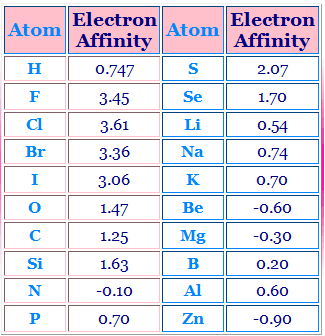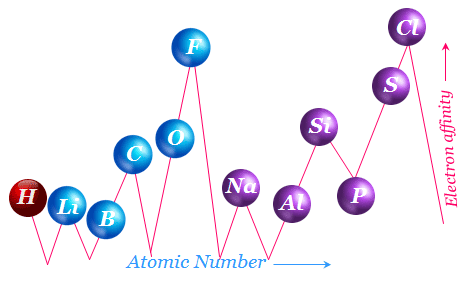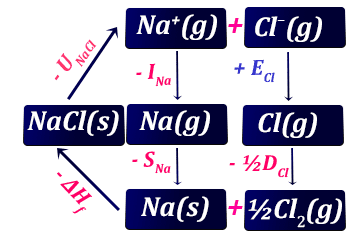# Electron Affinity

Electron Affinity of an element is defined as the amount of energy released when an electron is added to a neutral gaseous atom in its lowest energy state that is, ground state to convert it into uni-negative gaseous ion.
In simple words, the electron affinity of an atom is defined as the energy liberated when a gaseous atom captures an electron.
 A(g) + Electron→A- (g) + Electron Affinity
Evidently, electron affinity is equal in magnitude to the ionization energy of the species formed. The definition of electron affinity as the exothermicity of the electron capture reaction would lead to the negative sign according to our usual thermodynamic convention. But electron affinity is normally described with a positive sign.
Negative electron affinities are known. These negative values indicate that the species concerned does not like to have any more electron.

### Measurement of Electron Affinity

Electron affinities are difficult to obtain. These are often obtained from indirect measurements, by analysis of Born-Haber energy cycles in which one step is electron capture or by the direct study of electron capture from heated filaments.

### Factors Influencing Electron Affinity

The magnitude of Electron Affinity (EA) is influenced by the following factors such as,
1. Atomic Size.
2. Effective Nuclear Charge.
3. Electronic Configuration.

#### Electron affinity vs atomic Size

Larger the atomic radius lesser the tendency of the atom to attract the additional electron towards itself and lesser is the force of attraction exerted by the nucleus on the extra electron being added to the valence shell of the atom.
Thus Electron Affinity values decrease with increases atomic radius.

#### Electron affinity vs effective nuclear charge

Higher the magnitude of effective nuclear charge (Zeff) towards the periphery of an atom greater is the tendency of the atom to attract the additional electron towards itself.
Again greater the force of attraction exerted by the nucleus on the extra electron being added to the valence shell of the atom. As a result, higher the energy released when an extra electron is added to form an anion. Thus the magnitude of Electron Affinity of an atom increases with increasing Zeff value.

#### Electron affinity vs electronic configuration

nS², nS², nP⁶, nS², nP⁶
valence shell configuration posses very low electron affinity value due to having their stable valence shell configuration.Electron affinity of  different elements

### Electron affinity trend

#### Electron affinity across a group

In a group when move from top to bottom the size of atom generally increases with increasing atomic number and consequently, the magnitude of electron affinity decreases in the same direction.
There are some exceptions to this general rule as is evident from the following examples:
Although the elements of the second period of the periodic table are relatively smaller in size than those of the third-period elements, the electron affinity values of elements of the second period are smaller than the electron affinity values of third-period elements.
This unexpected behavior is explained by saying that the much smaller sizes of the second-period elements give a very much higher value of charge densities for the respective negative ions. A high value of electron density is opposed by the interelectronic repulsion forces.
Electron Affinity of fluorine is lower than that of Chlorine. The lower values of electron affinity for Florine due to the electron-electron repulsion in relatively compact 2P-Orbital of Florine atom. Thus the electron affinity values of halogens are:
 F Cl Br I - 3.6 eV - 3.8 eV - 3.5 eV - 3.2 eV

#### Electron affinity across a period

In a period, when we move from left to right Zeff value towards the new coming electron gradually increases with the increasing atomic number and hence the electron affinity gradually increases in the same direction.
A plot of electron affinities of elements up-to chlorine against atomic number shown as,Electron affinities as functions of atomic number

### Electron affinity questions answers

• Question
Calculate the electron affinity of chlorine from the Born - Haber cycle, given the following date : Lattice Energy = - 774 kJ mol⁻¹ , Ionization Potential of Na = 495 kJ mol⁻¹, Heat of Sublimation of Na = 108 kJ mol⁻¹, Energy for Bond Dissociation of chlorine (Cl2) = 240 kJ mol⁻¹ and Heat of Formation of NaCl = 410 kJ mol⁻¹.
• Answer
Born - Haber Cycle for the formation of NaCl (S) is:Born Haber Cycle
From the above Born - Haber cycle we can write as,

-UNaCl - INa + ECl - SNa - ½DCl - ΔHf = 0

or, ECl = UNaCl+INa+ SNa +½DCl + ΔHf

∴ ECl = -774 + 495 + 108 + 120 + 410

= 359 kJ mol-1
• Question
Account for the large decrees in electron affinity from lithium to beryllium despite the increase in nuclear charge.
• Answer
Atomic number and the electronic distribution of lithium and beryllium are:
Li    3    1S² 2S¹

Be    4    1S²2S²
Lithium has an incompletely filled 2S sub-shell while beryllium has the subshell filled. Thus lithium can receive an electron in its 2S sub-shell but for beryllium, a still higher energy 2P level has to be made of.
A filled shell or sub-shell leads to some extra stability. Hence beryllium resists an extra electron more than does lithium.
• Question
Why electron affinity of Nitrogen is - 0.10 eV while that of Phosphorus is + 0.70 eV?
• Answer
Atomic number and the electronic configuration of Nitrogen and Phosphorus are
N    7    1S²2S²2P³

P    15    1S²2S²2P⁶3S²3P³
Due to the smaller size of Nitrogen atom when an extra electron is added to the stable half - filled 2Porbital some amount of energy is required and hence electron affinity of nitrogen is negative.
On the other hand, due to the bigger size of P atom in compare to nitrogen less amount of energy released when the extra electron is added to the stable half - filled 3P orbitals and thus electron affinity of Phosphorus is expressed with a positive sign.
• Question
Explain why the electron affinity of chlorine is more than fluorine?
• Answer
The halogen possesses large electron affinity values indicating their strong tendency to form anions. This is easily understandable because their electronic configurations are only one electron short of the next noble gas element.
The electron affinity of chlorine is greater than that of F. This is probably due to the very small size of F atom which results in a very high charge density and consequently strong repulsion between existing valence shell electrons and the entering electron (new coming electron).
On the other hand, Cl being a bigger size, charge density is small and thus such repulsion is not strong enough. Hence the electron affinity Cl is greater than that of F.
• Question
Why electron gain enthalpy of Be and Mg are almost zero?
• Answer
Be and Mg have their electron affinity values equal to almost zero. Since Be and Mg have completely filled S orbitals.
Be      4   1S² 2S²

Mg   12   1S² 2S² 2P⁶ 3S²
The additional electron will be entering the 2P-orbital in the case of Be and 3P-orbitals in the case of Mg which are of considerably higher energy than the 2S- Orbitals respectively.
• Problem 6:
Why the electron affinity of noble gases is zero?
• Answer:
Inert gases in which the nS and nP orbitals are completely filled (nS² nP⁶ configuration) the incoming electron must go into an electron shall have the larger values for the principal quantum number, n. Thus inert gas has its electron affinity values equal to zero.
More Questions answer on inorganic chemistry

What is Electron Affinity? Measurement and Factors Influencing the magnitude of Electron Affinity and Periodic Variations with problems and their solutions

## Inorganic Chemistry

[Inorganic chemistry][column1]
[blogger]

Name

Email *

Message *